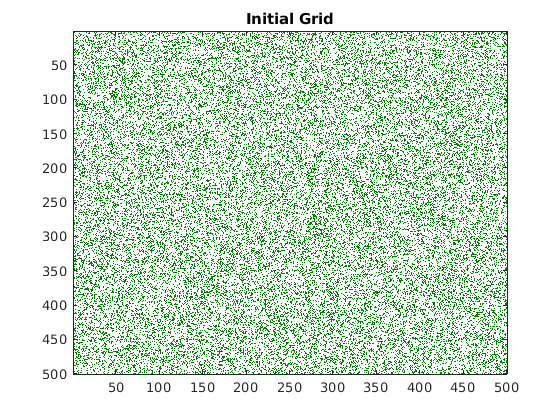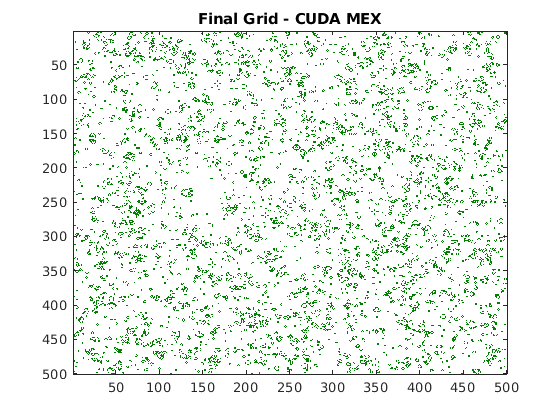# Stencil Processing on GPU

This example shows how to generate CUDA® kernels for stencil type operations by implementing "Game of Life" by John H. Conway.

"Game of Life" is a zero-player cellular automaton game that consists of a collection of cells (population) in a rectangular grid (universe). The cells evolve at discrete time steps known as generations. A set of mathematical rules applied to the cells and its neighbors control their life, death,and reproduction. This "Game of Life" implementation is based on the example provided in the e-book Experiments in MATLAB by Cleve Moler. The implementation follows these rules:

• Cells are arranged in a 2-D grid.

• At each step, the vitality of the eight nearest neighbors of each cell determines its fate.

• Any cell with exactly three live neighbors comes to life at the next step.

• A live cell with exactly two live neighbors remains alive at the next step.

• All other cells (including those with more than three neighbors) die at the next step or remain empty.

Here are some examples of how a cell is updated.Many array operations can be expressed as a stencil operation, where each element of the output array depends on a small region of the input array. The stencil in this example is the 3-by-3 region around each cell. Finite differences, convolution, median filtering, and finite-element methods are examples of other operations that stencil processing can perform.

### Prerequisites

• CUDA® enabled NVIDIA® GPU with compute capability 3.2 or higher.

• NVIDIA CUDA toolkit and driver.

• Environment variables for the compilers and libraries. For information on the supported versions of the compilers and libraries, see Third-party Products. For setting up the environment variables, see Setting Up the Prerequisite Products.

### Verify GPU Environment

To verify that the compilers and libraries necessary for running this example are set up correctly, use the `coder.checkGpuInstall` function.

```envCfg = coder.gpuEnvConfig('host'); envCfg.BasicCodegen = 1; envCfg.Quiet = 1; coder.checkGpuInstall(envCfg); ```

### Generate a Random Initial Population

Being that the game is zero-player, the evolution of the game is determined by its initial state. For this example, an initial population of cells is created on a two-dimensional grid with approximately 25% of the locations being alive.

```gridSize = 500; numGenerations = 100; initialGrid = (rand(gridSize,gridSize) > .75); % Draw the initial grid imagesc(initialGrid); colormap([1 1 1;0 0.5 0]); title('Initial Grid'); ```### Play the Game of Life

The gameoflife_orig.m function is a fully vectorized implementation of "Game of Life". The function updates all cells on the grid in one pass per their generation.

```type gameoflife_orig ```
```%% MATLAB vectorized implementation function grid = gameoflife_orig(initialGrid) % Copyright 2016-2019 The MathWorks, Inc. numGenerations = 100; grid = initialGrid; [gridSize,~] = size(initialGrid); % Loop through each generation updating the grid and displaying it. for generation = 1:numGenerations grid = updateGrid(grid, gridSize); imagesc(grid); colormap([1 1 1;0 0.5 0]); title(['Grid at Iteration ',num2str(generation)]); drawnow; end function X = updateGrid(X, N) % Index vectors increase or decrease the centered index by one % thereby accessing neighbors to the left,right,up, and down. p = [1 1:N-1]; q = [2:N N]; % Count how many of the eight neighbors are alive. neighbors = X(:,p) + X(:,q) + X(p,:) + X(q,:) + ... X(p,p) + X(q,q) + X(p,q) + X(q,p); % A live cell with two live neighbors, or any cell with % three live neighbors, is alive at the next step. X = (X & (neighbors == 2)) | (neighbors == 3); end end ```

Play the game by calling the `gameoflife_orig` function with an initial population. The game iterates through 100 generations and displays the population at each generation.

```gameoflife_orig(initialGrid); ```### Convert the Game of Life for GPU Code Generation

Looking at the calculations in the `updateGrid` function, it is apparent that the same operations are applied at each grid location independently. However, each cell must know about its eight neighbors. The modified gameoflife_stencil.m function uses the `gpucoder.stencilKernel` pragma to compute a 3-by-3 region around each cell. The GPU Coder™ implementation of the stencil kernel computes one element of the grid in each thread and uses shared memory to improve memory bandwidth and data locality.

```type gameoflife_stencil ```
```function grid = gameoflife_stencil(initialGrid) %#codegen % Copyright 2016-2019 The MathWorks, Inc. numGenerations = 100; grid = initialGrid; % Loop through each generation updating the grid. for generation = 1:numGenerations grid = gpucoder.stencilKernel(@updateElem, grid, [3,3], 'same'); end end function X = updateElem(window) [winH, winW] = size(window); neighbors = 0; for ww = 1:winW for wh = 1:winH neighbors = window(1,1) + window(1,2) + window(1,3) ... + window(2,1) + window(2,3) ... + window(3,1) + window(3,2) + window(3,3); end end X = (window(2,2) & (neighbors == 2)) | (neighbors == 3); end ```

### Generate CUDA MEX for the Function

To generate CUDA MEX for the `gameoflife_stencil` function, create a GPU code configuration object, and then use the `codegen` command.

```cfg = coder.gpuConfig('mex'); evalc('codegen -config cfg -args {initialGrid} gameoflife_stencil'); ```

### Run the MEX Function

Run the generated `gameoflife_stencil_mex` with the random initial population.

```gridGPU = gameoflife_stencil_mex(initialGrid); % Draw the grid after 100 generations imagesc(gridGPU); colormap([1 1 1;0 0.5 0]); title('Final Grid - CUDA MEX'); ```### Conclusion

CUDA code was generated for a simple stencil operation, Conway's "Game of Life". Implementation was accomplished by using the `gpucoder.stencilKernel` pragma. You can use the technique shown in this example to implement a range of stencil operations, including finite-element algorithms, convolutions, and filters.